Thermodynamics and Propulsion
Subsections

# 3.7 Brayton Cycle

[VW, S & B: 9.8-9.9, 9.12]

The Brayton cycle (or Joule cycle) represents the operation of a gas turbine engine. The cycle consists of four processes, as shown in Figure 3.13 alongside a sketch of an engine:

• a - b Adiabatic, quasi-static (or reversible) compression in the inlet and compressor;
• b - c Constant pressure fuel combustion (idealized as constant pressure heat addition);
• c - d Adiabatic, quasi-static (or reversible) expansion in the turbine and exhaust nozzle, with which we
1. take some work out of the air and use it to drive the compressor, and
2. take the remaining work out and use it to accelerate fluid for jet propulsion, or to turn a generator for electrical power generation;
• d - a Cool the air at constant pressure back to its initial condition.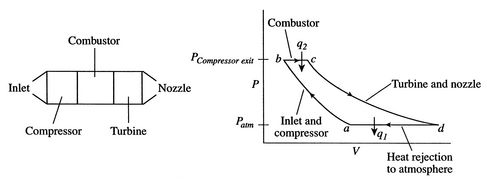The components of a Brayton cycle device for jet propulsion are shown in Figure 3.14. We will typically represent these components schematically, as in Figure 3.15. In practice, real Brayton cycles take one of two forms. Figure 3.16(a) shows an open'' cycle, where the working fluid enters and then exits the device. This is the way a jet propulsion cycle works. Figure 3.16(b) shows the alternative, a closed cycle, which recirculates the working fluid. Closed cycles are used, for example, in space power generation.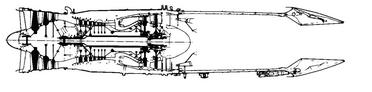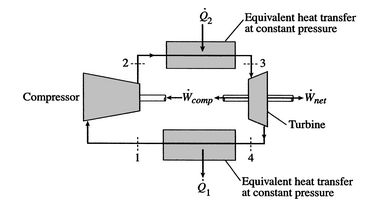[Open cycle operation][Closed cycle operation]Muddy Points

Would it be practical to run a Brayton cycle in reverse and use it as refrigerator? (MP 3.10)

## 3.7.1 Work and Efficiency

The objective now is to find the work done, the heat absorbed, and the thermal efficiency of the cycle. Tracing the path shown around the cycle from--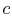-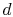and back to, the first law gives (writing the equation in terms of a unit mass),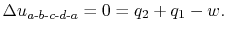Hereis zero becauseis a function of state, and any cycle returns the system to its starting state3.2. The net work done is therefore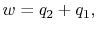where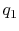,are defined as heat received by the system (is negative). We thus need to evaluate the heat transferred in processes-and-.

For a constant pressure, quasi-static process the heat exchange per unit mass isWe can see this by writing the first law in terms of enthalpy (see Section 2.3.4) or by remembering the definition of.

The heat exchange can be expressed in terms of enthalpy differences between the relevant states. Treating the working fluid as a perfect gas with constant specific heats, for the heat addition from the combustor,The heat rejected is, similarly,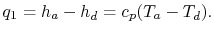The net work per unit mass is given byThe thermal efficiency of the Brayton cycle can now be expressed in terms of the temperatures:(3..8)

To proceed further, we need to examine the relationships between the different temperatures. We know that pointsandare on a constant pressure process as are pointsand, and;. The other two legs of the cycle are adiabatic and reversible, soTherefore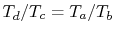, or, finally,. Using this relation in the expression for thermal efficiency, Eq. (3.8) yields an expression for the thermal efficiency of a Brayton cycle:(3..9)

The temperature ratio across the compressor,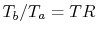. In terms of compressor temperature ratio, and using the relation for an adiabatic reversible process we can write the efficiency in terms of the compressor (and cycle) pressure ratio, which is the parameter commonly used:(3..10)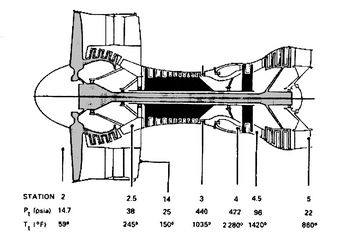Figure 3.17 shows pressures and temperatures through a gas turbine engine (the PW4000, which powers the 747 and the 767).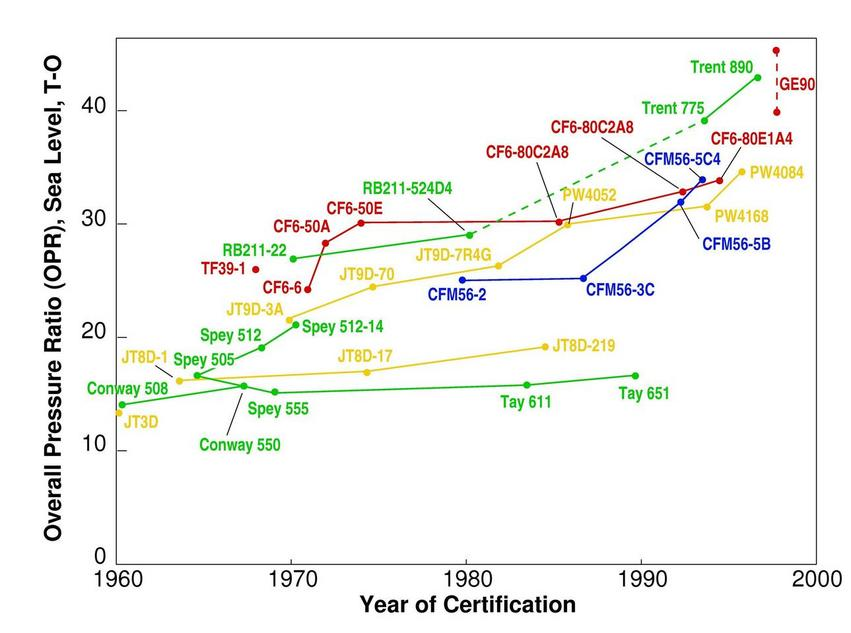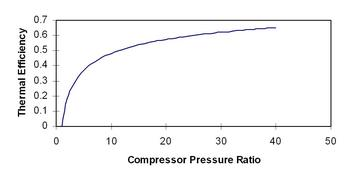Equation (3.10) says that for a high cycle efficiency, the pressure ratio of the cycle should be increased. This trend is plotted in Figure 3.19. Figure 3.18 shows the history of aircraft engine pressure ratio versus entry into service, and it can be seen that there has been a large increase in cycle pressure ratio. The thermodynamic concepts apply to the behavior of real aerospace devices!

Muddy Points

When flow is accelerated in a nozzle, doesn't that reduce the internal energy of the flow and therefore the enthalpy? (MP 3.11)

Why do we say the combustion in a gas turbine engine is constant pressure? (MP 3.12)

Why is the Brayton cycle less efficient than the Carnot cycle? (MP 3.13)

If the gas undergoes constant pressure cooling in the exhaust outside the engine, is that still within the system boundary? (MP 3.14)

Does it matter what labels we put on the corners of the cycle or not? (MP 3.15)

Is the work done in the compressor always equal to the work done in the turbine plus work out (for a Brayton cyle)? (MP 3.16)

## 3.7.2 Gas Turbine Technology and Thermodynamics

The turbine entry temperature,, is fixed by materials technology and cost. (If the temperature is too high, the blades fail.) Figures 3.20 and 3.21 show the progression of the turbine entry temperatures in aeroengines. Figure 3.20 is from Rolls Royce and Figure 3.21 is from Pratt & Whitney. Note the relation between the gas temperature coming into the turbine blades and the blade melting temperature.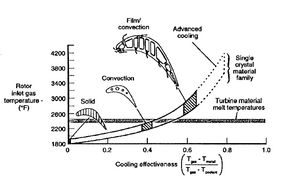For a given level of turbine technology (in other words given maximum temperature) a design question is what should the compressorbe? What criterion should be used to decide this? Maximum thermal efficiency? Maximum work? We examine this issue below.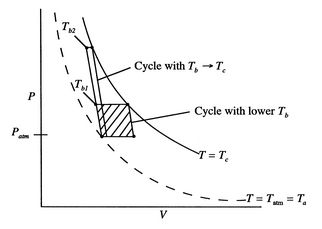The problem is posed in Figure 3.22, which shows two Brayton cycles. For maximum efficiency we would likeas high as possible. This means that the compressor exit temperature approaches the turbine entry temperature. The net work will be less than the heat received; asthe heat received approaches zero and so does the net work.

The net work in the cycle can also be expressed as, evaluated in traversing the cycle. This is the area enclosed by the curves, which is seen to approach zero as.

The conclusion from either of these arguments is that a cycle designed for maximum thermal efficiency is not very useful in that the work (power) we get out of it is zero.

A more useful criterion is that of maximum work per unit mass (maximum power per unit mass flow). This leads to compact propulsion devices. The work per unit mass is given by: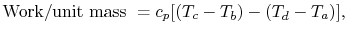whereis the maximum turbine inlet temperature (a design constraint) andis atmospheric temperature. The design variable is the compressor exit temperature,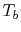, and to find the maximum as this is varied, we differentiate the expression for work with respect to: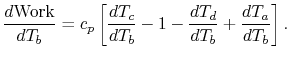The first and the fourth terms on the right hand side of the above equation are both zero (the turbine entry temperature is fixed, as is the atmospheric temperature). The maximum work occurs where the derivative of work with respect tois zero:(3..11)

To use Eq. (3.11), we need to relate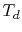and. We know thatHence,Plugging this expression for the derivative into Eq. (3.11) gives the compressor exit temperature for maximum work as. In terms of temperature ratio,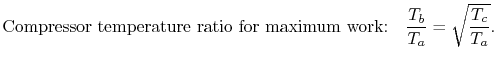The condition for maximum work in a Brayton cycle is different than that for maximum efficiency. The role of the temperature ratio can be seen if we examine the work per unit mass which is delivered at this condition: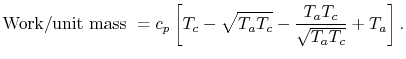Ratioing all temperatures to the engine inlet temperature,To find the power the engine can produce, we need to multiply the work per unit mass by the mass flow rate:(3..12)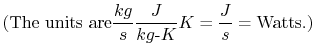The trend of work output vs. compressor pressure ratio, for different temperature ratios, is shown in Figure 3.23.[Gas turbine engine core]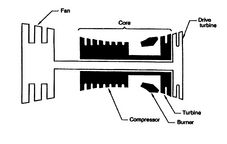[Core power vs. turbine entry temperature]Figure 3.24 shows the expression for power of an ideal cycle compared with data from actual jet engines. Figure 3.24(a) shows the gas turbine engine layout including the core (compressor, burner, and turbine). Figure 3.24(b) shows the core power for a number of different engines as a function of the turbine rotor entry temperature. The equation in the figure for horsepower (HP) is the same as that which we just derived, except for the conversion factors. The analysis not only shows the qualitative trend very well but captures much of the quantitative behavior too.

A final comment (for this section) on Brayton cycles concerns the value of the thermal efficiency. The Brayton cycle thermal efficiency contains the ratio of the compressor exit temperature to atmospheric temperature, so that the ratio is not based on the highest temperature in the cycle, as the Carnot efficiency is. For a given maximum cycle temperature, the Brayton cycle is therefore less efficient than a Carnot cycle.

Muddy Points

What are the units of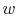in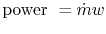? (MP 3.17)

Question about the assumptions made in the Brayton cycle for maximum efficiency and maximum work (MP 3.18)

You said that for a gas turbine engine modeled as a Brayton cycle the work done is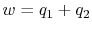, whereis the heat added andis the heat rejected. Does this suggest that the work that you get out of the engine doesn't depend on how good your compressor and turbine are?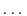since the compression and expansion were modeled as adiabatic. (MP 3.19)

## 3.7.3 Brayton Cycle for Jet Propulsion: the Ideal Ramjet

A schematic of a ramjet is given in Figure 3.25.In the ramjet there are no moving parts.'' The processes that occur in this propulsion device are:

•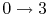: Isentropic diffusion (slowing down) and compression, with a decrease in Mach number,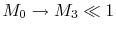.
•: Constant pressure combustion.
•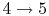: Isentropic expansion through the nozzle.

The ramjet thermodynamic cycle efficiency can be written in terms of flight Mach number,, as follows:and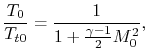so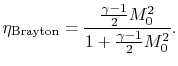Muddy Points

Why don't we like the numbers 1 and 2 for the stations? Why do we go 0-3? (MP 3.20)

For the Brayton cycle efficiency, why does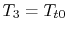? (MP 3.21)

## 3.7.4 MIT Cogenerator

MIT operates a Brayton cycle power generator on campus. For more information, see the website at https://cogen.mit.edu/ctg.cfm .

UnifiedTP